# Linear Shunt DC-DC Converter

The alternative for a linear series voltage converter is a linear shunt voltage converter. The principle of operation for this type of DC-DC converter is shown in Fig. 1 (a).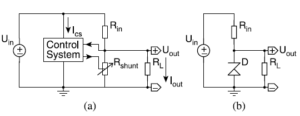Fig. 1: The principle of a linear shunt voltageconverter and (b) a simple practical implementation

Uin is lowered to Uout by means of the resistive division between the fixed input resistor Rin and both the load RL and the variable shunt resistor Rshunt , where Uout is calculated through as follows :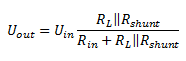Rin can either be the intrinsic output resistance of  Uin, an added resistor or the combination of both.

Uout is kept constant under varying RL and Uin conditions by adapting the value of Rshunt.

This operation can be performed by a control system, providing feedback from Uout. The control system consumes a certain amount of power by drawing a current Ics from Uin or Uout. The fact that  Uout can also be directly used to supply the control systems is due to the self-starting nature of this circuit, as opposed to the linear series  voltage converter.

The voltage used for supplying the control system will depend on whether Uout  has a sufficiently large value. In the following analysis it is assumed that Uin is used for this purpose, for which the results merely differ little from the other possibility.

Feedback of Uout is however not always required, as illustrated by the simple practical implementation of Fig. 1(b). For this implementation the shunt resistor is replaced by a reverse-biased zener diode D. In this way a quasi constant Uout can be achieved, if the current through D is kept large enough for it to operate in the zener-region.

For a shunt converter ηlin  is calculated as below :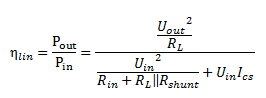Fig. 2 graphically illustrates  ηlin as a function of the output power  Pout, for a constant voltage conversion ratio  klin.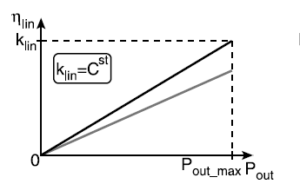Fig.2

The black curve is valid for the ideal case, where  Ics is zero and the gray curve is valid for a finite non-zero Ics. As opposed to a linear series converter,  ηlin is intrinsically linear dependent on Pout . It can be seen that  ηlin is zero for  Pout = 0 and that it has a maximal value equal to  klin, occurring at the maximal output power  Pout_max which is given below :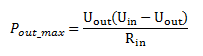For a given  Uin and Uout, Pout_max is determined by the inverse of the value of  Rin.

Unlike a linear series converter, where ηlin is ideally independent of Pout, a linear shunt converter only achieves its maximal  ηlin at Pout_maxThis behavior makes a linear shunt converter inferior compared to a series converter, in terms of  ηlin.

However, its simple practical implementation makes it suitable for applications that require a small and quasi constant Pout .

Furthermore, a linear shunt converter can prove to be more practical than a linear series converter in applications that have a low value for klin and ηlin.

In such a case the voltage difference Uin − Uout  will only be present over the passive resistor Rin rather than over an active device, of which the maximal voltage is limited.

The simple nature of a linear shunt voltage converter, and its lack of large passives, makes it suitable for monolithic integration in non-critical applications.

Obviously, the problem of on-chip power dissipation remains and becomes more limiting than for linear series voltage converters.